Test: Reasoning Ability - 1

# Test: Reasoning Ability - 1 - Banking Exams

Test Description

## 20 Questions MCQ Test IBPS PO Mock Test Series & Past Year Papers - Test: Reasoning Ability - 1

Test: Reasoning Ability - 1 for Banking Exams 2023 is part of IBPS PO Mock Test Series & Past Year Papers preparation. The Test: Reasoning Ability - 1 questions and answers have been prepared according to the Banking Exams exam syllabus.The Test: Reasoning Ability - 1 MCQs are made for Banking Exams 2023 Exam. Find important definitions, questions, notes, meanings, examples, exercises, MCQs and online tests for Test: Reasoning Ability - 1 below.
Solutions of Test: Reasoning Ability - 1 questions in English are available as part of our IBPS PO Mock Test Series & Past Year Papers for Banking Exams & Test: Reasoning Ability - 1 solutions in Hindi for IBPS PO Mock Test Series & Past Year Papers course. Download more important topics, notes, lectures and mock test series for Banking Exams Exam by signing up for free. Attempt Test: Reasoning Ability - 1 | 20 questions in 10 minutes | Mock test for Banking Exams preparation | Free important questions MCQ to study IBPS PO Mock Test Series & Past Year Papers for Banking Exams Exam | Download free PDF with solutions
 1 Crore+ students have signed up on EduRev. Have you?
Test: Reasoning Ability - 1 - Question 1

### Directions: Study the following information carefully and answer the questions given below. A certain number of boxes are placed one above the other. Only one box is kept between Box X and Box Z, which is placed immediately above or below Box B. Box R is placed sixth from the bottom of the stack. Five boxes are kept between Box R and Box P, which is not the topmost box. Box S is kept either immediately above or immediately below Box P. As many boxes are kept between Box S and Box R as between Box R and Box K. Three boxes are kept between Box B and Box K and both the boxes are kept below Box R. As many boxes are placed above Box S as below Box Z. Box Q is kept exactly between Box P and Box R. Q. Which of the following boxes occupies the bottom most position?

Detailed Solution for Test: Reasoning Ability - 1 - Question 1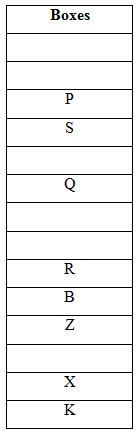Box R is placed sixth from the bottom of the stack. Five boxes are kept between Box R and Box P, which is not the topmost box. Box S is kept either immediately above or immediately below Box P. Box Q is kept exactly between Box P and Box R.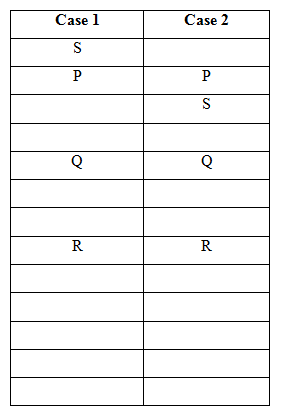Three boxes are kept between Box B and Box K and both the boxes are kept below Box R. As many boxes are kept between Box S and Box R as between Box R and Box K.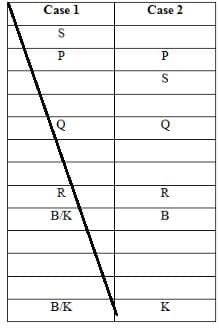Since Box K cannot be placed, Case 1 gets cancelled.

Only one box is kept between Box X and Box Z, which is placed immediately above or below Box B. As many boxes are placed above Box S as below Box Z.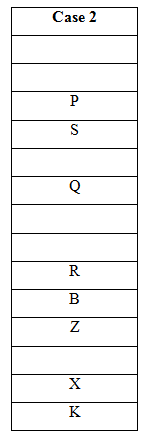Test: Reasoning Ability - 1 - Question 2

### Directions: Study the following information carefully and answer the questions given below. A certain number of boxes are placed one above the other. Only one box is kept between Box X and Box Z, which is placed immediately above or below Box B. Box R is placed sixth from the bottom of the stack. Five boxes are kept between Box R and Box P, which is not the topmost box. Box S is kept either immediately above or immediately below Box P. As many boxes are kept between Box S and Box R as between Box R and Box K. Three boxes are kept between Box B and Box K and both the boxes are kept below Box R. As many boxes are placed above Box S as below Box Z. Box Q is kept exactly between Box P and Box R. Q. How many boxes are there in the above arrangement?

Detailed Solution for Test: Reasoning Ability - 1 - Question 2Box R is placed sixth from the bottom of the stack. Five boxes are kept between Box R and Box P, which is not the topmost box. Box S is kept either immediately above or immediately below Box P. Box Q is kept exactly between Box P and Box R.Three boxes are kept between Box B and Box K and both the boxes are kept below Box R. As many boxes are kept between Box S and Box R as between Box R and Box K.Since Box K cannot be placed, Case 1 gets cancelled.

Only one box is kept between Box X and Box Z, which is placed immediately above or below Box B. As many boxes are placed above Box S as below Box Z.Test: Reasoning Ability - 1 - Question 3

### Directions: Study the following information carefully and answer the questions given below. A certain number of boxes are placed one above the other. Only one box is kept between Box X and Box Z, which is placed immediately above or below Box B. Box R is placed sixth from the bottom of the stack. Five boxes are kept between Box R and Box P, which is not the topmost box. Box S is kept either immediately above or immediately below Box P. As many boxes are kept between Box S and Box R as between Box R and Box K. Three boxes are kept between Box B and Box K and both the boxes are kept below Box R. As many boxes are placed above Box S as below Box Z. Box Q is kept exactly between Box P and Box R. Q. Which of the following statements is true with respect to the above arrangement?

Detailed Solution for Test: Reasoning Ability - 1 - Question 3Box R is placed sixth from the bottom of the stack. Five boxes are kept between Box R and Box P, which is not the topmost box. Box S is kept either immediately above or immediately below Box P. Box Q is kept exactly between Box P and Box R.Three boxes are kept between Box B and Box K and both the boxes are kept below Box R. As many boxes are kept between Box S and Box R as between Box R and Box K.Since Box K cannot be placed, Case 1 gets cancelled.

Only one box is kept between Box X and Box Z, which is placed immediately above or below Box B. As many boxes are placed above Box S as below Box Z.Test: Reasoning Ability - 1 - Question 4

Directions: Study the following information carefully and answer the questions given below.

A certain number of boxes are placed one above the other. Only one box is kept between Box X and Box Z, which is placed immediately above or below Box B. Box R is placed sixth from the bottom of the stack. Five boxes are kept between Box R and Box P, which is not the topmost box. Box S is kept either immediately above or immediately below Box P. As many boxes are kept between Box S and Box R as between Box R and Box K. Three boxes are kept between Box B and Box K and both the boxes are kept below Box R. As many boxes are placed above Box S as below Box Z. Box Q is kept exactly between Box P and Box R.

Q. Which of the following boxes occupies the bottom most position?

Detailed Solution for Test: Reasoning Ability - 1 - Question 4Box R is placed sixth from the bottom of the stack. Five boxes are kept between Box R and Box P, which is not the topmost box. Box S is kept either immediately above or immediately below Box P. Box Q is kept exactly between Box P and Box R.Three boxes are kept between Box B and Box K and both the boxes are kept below Box R. As many boxes are kept between Box S and Box R as between Box R and Box K.Since Box K cannot be placed, Case 1 gets cancelled.

Only one box is kept between Box X and Box Z, which is placed immediately above or below Box B. As many boxes are placed above Box S as below Box Z.Test: Reasoning Ability - 1 - Question 5

Directions: Study the following information carefully and answer the questions given below.

A certain number of boxes are placed one above the other. Only one box is kept between Box X and Box Z, which is placed immediately above or below Box B. Box R is placed sixth from the bottom of the stack. Five boxes are kept between Box R and Box P, which is not the topmost box. Box S is kept either immediately above or immediately below Box P. As many boxes are kept between Box S and Box R as between Box R and Box K. Three boxes are kept between Box B and Box K and both the boxes are kept below Box R. As many boxes are placed above Box S as below Box Z. Box Q is kept exactly between Box P and Box R.

Q. Which of the following pair represents the boxes that are placed immediately above and below Box B respectively?

Detailed Solution for Test: Reasoning Ability - 1 - Question 5Box R is placed sixth from the bottom of the stack. Five boxes are kept between Box R and Box P, which is not the topmost box. Box S is kept either immediately above or immediately below Box P. Box Q is kept exactly between Box P and Box R.Three boxes are kept between Box B and Box K and both the boxes are kept below Box R. As many boxes are kept between Box S and Box R as between Box R and Box K.Since Box K cannot be placed, Case 1 gets cancelled.

Only one box is kept between Box X and Box Z, which is placed immediately above or below Box B. As many boxes are placed above Box S as below Box Z.Test: Reasoning Ability - 1 - Question 6

Directions: In each of the following questions, relationship between different elements is shown in the statements followed by two conclusions. Find the conclusion which is definitely true.

a) If only conclusion I follows.

b) If only conclusion II follows.

c) If either conclusion I or II follows.

d) If neither conclusion I nor II follows.

e) If both conclusions I and II follow.

Statements:

K > L ≤ O = P; S ≤ L < Q

Conclusions:

I). S ≤ P

II). Q > K

Detailed Solution for Test: Reasoning Ability - 1 - Question 6

I). S ≤ P (S ≤ L ≤ O = P) → True

II). Q > K (Q > L < K) → False

So, only conclusion I follows.

Test: Reasoning Ability - 1 - Question 7

Directions: In each of the following questions, relationship between different elements is shown in the statements followed by two conclusions. Find the conclusion which is definitely true.

a) If only conclusion I follows.

b) If only conclusion II follows.

c) If either conclusion I or II follows.

d) If neither conclusion I nor II follows.

e) If both conclusions I and II follow.

Statements:

G < I > H ≥ D; C < S ≤ Y = H

Conclusions:

I). S ≤ D

II). I ≥ S

Detailed Solution for Test: Reasoning Ability - 1 - Question 7

I). S ≤ D (S ≤ Y = H ≥ D) →False

II). I ≥ S (I > H = Y ≥ S) → False

So, neither conclusion I nor II follows.

Test: Reasoning Ability - 1 - Question 8

Directions: In each of the following questions, relationship between different elements is shown in the statements followed by two conclusions. Find the conclusion which is definitely true.

a) If only conclusion I follows.

b) If only conclusion II follows.

c) If either conclusion I or II follows.

d) If neither conclusion I nor II follows.

e) If both conclusions I and II follow.

Statements:

F ≤ M ≤ K = R; B ≤ F > N = J

Conclusions:

I). J < M

II). R ≥ B

Detailed Solution for Test: Reasoning Ability - 1 - Question 8

I). J < M (J = N < F ≤ M) → True

II). R ≥ B (R = K ≥ M ≥ F ≥ B) → True

So, both I and II follow.

Test: Reasoning Ability - 1 - Question 9

Directions: tudy the following information carefully and answer the questions given below.

In a certain coding language,

‘Street light star’ is written as ‘wa qu ks’

‘Hero star award’ is written as ‘tv wa pk’

‘Light house scene’ is written as ‘jh bl qu’

If ‘evening scene’ is coded as ‘bl nu’, then how is ‘evening’ coded in the above language?

Detailed Solution for Test: Reasoning Ability - 1 - Question 9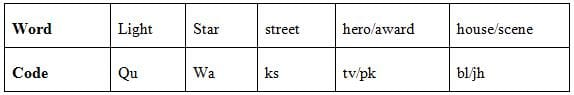Test: Reasoning Ability - 1 - Question 10

Directions: Study the following information carefully and answer the questions given below.

In a certain coding language,

‘Street light star’ is written as ‘wa qu ks’

‘Hero star award’ is written as ‘tv wa pk’

‘Light house scene’ is written as ‘jh bl qu’

What could be the possible code of ‘scene’ in the given coded language?

Detailed Solution for Test: Reasoning Ability - 1 - Question 10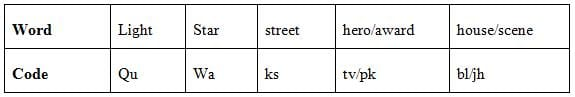Test: Reasoning Ability - 1 - Question 11

Directions: Study the following information carefully and answer the questions given below.

Arun walks 7m towards east and stops at point Q. From there, he turns 90° in anticlockwise direction and walks for 4m before stopping at S. Then he turns 135° in clockwise direction and walks for ‘x’ m before stopping at R which is exactly east of Q. From R, he walks 5m south and stops at V, from where he turns 90° in clockwise direction and walks 6m before he finally stops at Z, which is exactly south of S.

Q. What is the value of ‘x’?

Detailed Solution for Test: Reasoning Ability - 1 - Question 11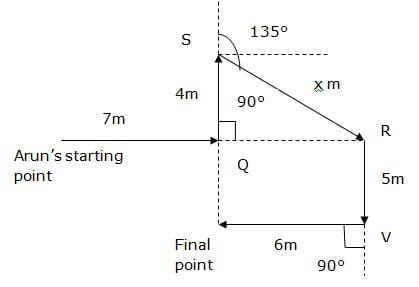Test: Reasoning Ability - 1 - Question 12

Directions: Study the following information carefully and answer the questions given below.

Arun walks 7m towards east and stops at point Q. From there, he turns 90° in anticlockwise direction and walks for 4m before stopping at S. Then he turns 135° in clockwise direction and walks for ‘x’ m before stopping at R which is exactly east of Q. From R, he walks 5m south and stops at V, from where he turns 90° in clockwise direction and walks 6m before he finally stops at Z, which is exactly south of S.

Q. Which of the following points lie in a straight line?

Detailed Solution for Test: Reasoning Ability - 1 - Question 12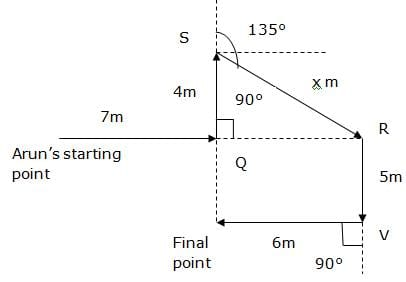Test: Reasoning Ability - 1 - Question 13

Directions: Study the following information carefully and answer the questions given below.

Arun walks 7m towards east and stops at point Q. From there, he turns 90° in anticlockwise direction and walks for 4m before stopping at S. Then he turns 135° in clockwise direction and walks for ‘x’ m before stopping at R which is exactly east of Q. From R, he walks 5m south and stops at V, from where he turns 90° in clockwise direction and walks 6m before he finally stops at Z, which is exactly south of S.

Q. In which direction is the starting point of Arun with respect to Z?

Detailed Solution for Test: Reasoning Ability - 1 - Question 13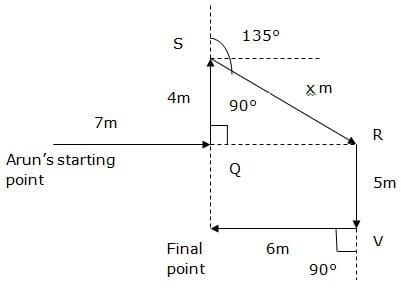Test: Reasoning Ability - 1 - Question 14

How many digits are there in the number ˜85721943™ which occupy the same position when the digits are arranged in ascending order from right to left?

Detailed Solution for Test: Reasoning Ability - 1 - Question 14

85721943

98754321

Test: Reasoning Ability - 1 - Question 15

How many such pairs of letters are there in the word ˜SYLLABUS™, each of which has as many letters between them in the word, as they have in the English alphabet (both in forward and backward directions)?

Detailed Solution for Test: Reasoning Ability - 1 - Question 15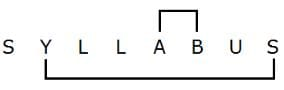Test: Reasoning Ability - 1 - Question 16

Directions: Study the following information carefully and answer the questions given below.

There are seven members in three generations of a family. The family has two married couple. S is the grandson of B, who has only one son. B is the mother-in-law of P. A and L are siblings of S. L is the granddaughter of C. A is the son of P. H is one of the family members. H is married to P.

Q. How is L related to P?

Detailed Solution for Test: Reasoning Ability - 1 - Question 16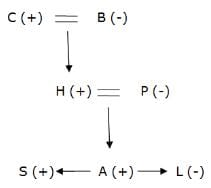Test: Reasoning Ability - 1 - Question 17

Directions: Study the following information carefully and answer the questions given below.

There are seven members in three generations of a family. The family has two married couple. S is the grandson of B, who has only one son. B is the mother-in-law of P. A and L are siblings of S. L is the granddaughter of C. A is the son of P. H is one of the family members. H is married to P.

Q. If B has one daughter, K. Then, how is K related to A?

Detailed Solution for Test: Reasoning Ability - 1 - Question 17Test: Reasoning Ability - 1 - Question 18

Directions: Study the following information carefully and answer the questions given below.

In a certain coding language,

‘very interesting is around’ is written as ‘mo tu pa ka’

‘biggest energy is succeed’ is written as ‘tu ne ga xl’

‘nature become very popular’ is written as ‘ki ta mo ab’

‘succeed is very interesting’ is written as ‘xl tu ka mo’

Q. What is the code for ‘very interesting’ in the above coding language?

Detailed Solution for Test: Reasoning Ability - 1 - Question 18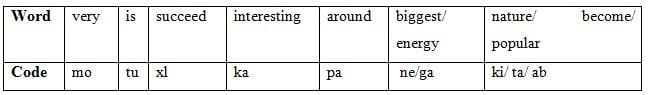Test: Reasoning Ability - 1 - Question 19

Q. If ‘world become popular’ is coded as ‘ah ta ki’, then what will be the code for ‘nature’?

Detailed Solution for Test: Reasoning Ability - 1 - Question 19Test: Reasoning Ability - 1 - Question 20

Directions: Study the following information carefully and answer the questions given below.

In a certain coding language,

‘very interesting is around’ is written as ‘mo tu pa ka’

‘biggest energy is succeed’ is written as ‘tu ne ga xl’

‘nature become very popular’ is written as ‘ki ta mo ab’

‘succeed is very interesting’ is written as ‘xl tu ka mo’

Q. Which of the following is the code for ‘energy’?

Detailed Solution for Test: Reasoning Ability - 1 - Question 20## IBPS PO Mock Test Series & Past Year Papers

33 docs|72 tests
Information about Test: Reasoning Ability - 1 Page
In this test you can find the Exam questions for Test: Reasoning Ability - 1 solved & explained in the simplest way possible. Besides giving Questions and answers for Test: Reasoning Ability - 1, EduRev gives you an ample number of Online tests for practice

33 docs|72 tests